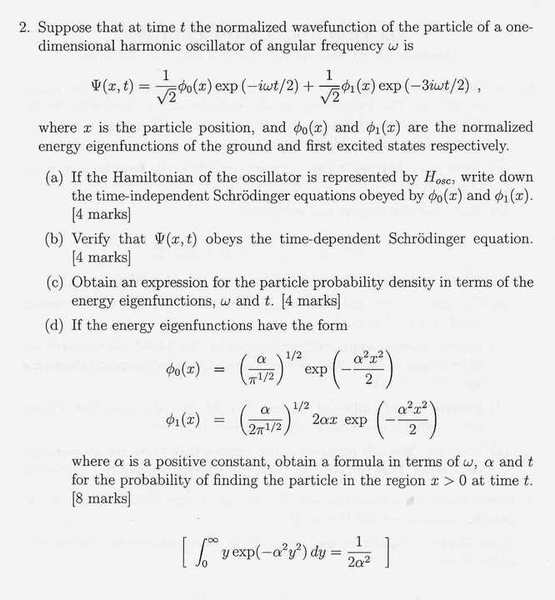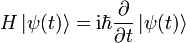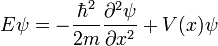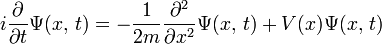# Quantum mechanics-help!

## Homework Statement

Below is a past paper question from a paper i am working through in preperation for my exams. I am having particular trouble with this question, it doesnt seem as straight forward and formulated as other questions. I am unsure if anything i have done is right so any help would be great.## Homework Equations

(a)Time independent equation(b)
Time dependent Schrodinger(c)
Probabilty Density $$=\left|\psi(x,t)\right|^{2}$$

## The Attempt at a Solution

a)

$$H_{osc}\phi_{0}(x)=E\phi_{0}(x)$$

$$\\\\H_{osc}\phi_{1}(x)=E\phi_{1}(x)$$

b)

I tried simply putting the wavefunction into the Time dependent Schrodinger equation and differentiating. This mustnt be the way since there is an m on the LHS.

c)

Probabilty Density

$$\left|\psi(x,t)\right|^{2}=1/2[\phi^{2}_{0}+\phi^{2}_{1}+\phi^{*}_{0}\phi_{1}exp(-i\omega t)+\phi^{*}_{1}\phi_{0}exp(i\omega t)]$$

d)

$$\int^{\infty}_{0}\left|\psi(x,t)\right|^{2}dx=1/2\int^{\infty}_{0}[\phi^{2}_{0}+\phi^{2}_{1}+\phi^{*}_{0}\phi_{1}exp(-i\omega t)+\phi^{*}_{1}\phi_{0}exp(i\omega t)]dx$$

^ This doesnt work out too well

The purpose of this problem is to show that the given combination of wave functions gives you an oscillating probability density going back and forth at the classical frequency. I don't have time to go through all your equations at the moment but item b, for one, ought to work out the way you've set it up. I think the dimensions are consistent between the LHS and the RHS.

Matterwave